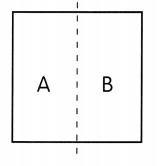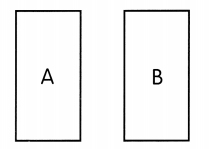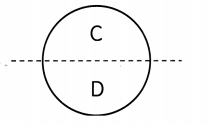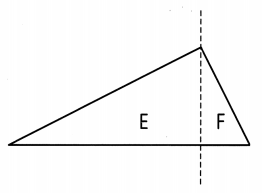# Math in Focus Grade 1 Chapter 5 Practice 2 Answer Key Exploring Plane Shapes

This handy Math in Focus Grade 1 Workbook Answer Key Chapter 5 Practice 2 Exploring Plane Shapes detailed solutions for the textbook questions.

## Math in Focus Grade 1 Chapter 5 Practice 2 Answer Key Exploring Plane Shapes

Question 1.
Josh has a square piece of paper. He folds it and unfolds it. Then he draws a line along the fold. Now he has two new shapes, A and B.Write yes or no.

a. Are Shape A and Shape B the same shape? ____
Yes, shape A and shape B both are having the same shape.

b. Are Shape A and Shape B the same size? ____
Yes, the shape A and shape B are the same size.

Count.

c. How many sides are there?
Shape A ___ Shape B ____
Shape A has 2 sides and shape B has 2 sides.

d. How many corners are there?
Shape A ___ Shape B ____
Shape A has 2 corners and shape B also have 2 corners.

Write yes or no.

e. Are Shape A and Shape B different?
No, the shape A and shape B both are same.

Josh then cuts out Shape A and Shape B.f. Can Shape A fit exactly over Shape B? ________
Yes, the shape A fits exactly over the shape B.

Are the shapes the same shape and size? Write yes or no.

Question 2.Shapes C and D ____